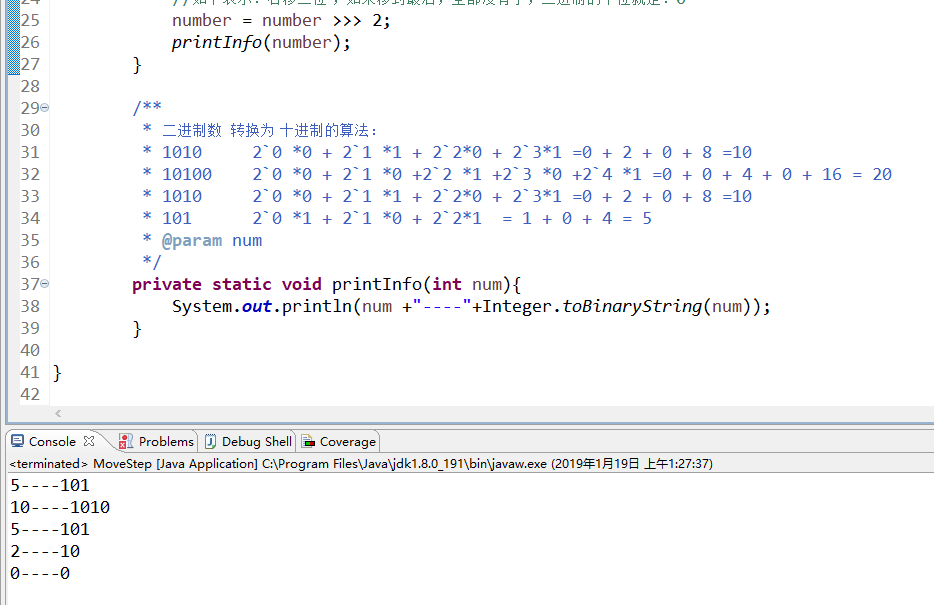• 10:10105:1012:101:1算法描述：首先用1去与一个数进行与运算，如果返回真说明这个数对应的二进制数的末尾为1，否则为0把得到结果存储到一个字符数组中，然后让这个数除以2，此时，尾数就是上次的倒数第二个数，重复...
10:1010 5:101 2:10 1:1算法描述：首先用1去与一个数进行与运算，如果返回真说明这个数对应的二进制数的末尾为1，否则为0  把得到结果存储到一个字符数组中，然后让这个数除以2，此时，尾数就是上次的倒数第二个数，  重复这个算法，直到这个数为0，退出循环。  得到的结果一定的这个数二进制的反序，此时只要写一个反序算法即可。关于位与运算：首先把两个操作数转换为二进制，而数在内存中就是以二进制存放（因此本算法效率极高），按对应的位进行比较  当且仅当两个操作数相同的位上的数都为1结果为1  比如   10：1010         &    7：0111    2：0010 10&7==2#define  _CRT_SECURE_NO_WARNINGS#include <stdio.h>#include <stdlib.h>int toBinary(int n, char *binary);void reverse(char *str, int length);void main(){ /*由于计算机和internet都没有将一个负转换为二进制数，将本算法稍经修改即可*/ int number=0; char binary = { 0 }; int length = 0; scanf("%d",&number); length = toBinary(number, binary); reverse(binary, length); printf("%d对应的二进制数：",number); puts(binary); system("pause");}//倒叙用位与运算取每一个二进制位int toBinary(int n, char *binary){ int temp = 1; int i = 0; while (n != 0) {  //核心算法  binary[i++] = (n & temp == 1) ? '1' : '0';  n /= 2; } binary[i] = 0; return i;}//数组反转算法void reverse(char *str, int length){ int i = 0; char temp; for (i = 0; i < length / 2; i++) {  temp = str[i];  str[i] = str[length - i - 1];  str[length - i - 1] = temp; } str[length] = 0;}
转载于:https://www.cnblogs.com/chaeyeon/p/6144438.html
展开全文• Java编写程序计算十进制整数及小数，转二进制
十进制转二进制怎么转？
首先来说一下十进制怎么转换成二进制，十进制转二进制就是初二取余，小数部分的是乘二取整。
比如一个十进制数是100
那么转换成二进制就是
100/2 =50 余0
50/2 =25 余0
25/2 =12 余1
12/2 =6 余0
6/2 =3 余0
3/2 =1 余1
1/2 =0 余1
因为二进制是反过来的，所以二进制就是1100100
小数部分是乘二取余
比如：10.25
那么先算整数部分
10/2=5 余0
5/2=2 余1
2/2=1 余0
1/2=0 余1
整数部分是1010
小数部分
0.25*2=0.5 整0
0.5*2=1.0 整1
因为到0为止，小数二进制不用取反，所以是01
合起来就是1010.01
根据以上的规律分析我们可以用代码来实现。
代码如下：
import java.util.Scanner;//因为下面用到的Scanner类，所以要引包

public class Test {
public static void main(String[] args) {
System.out.println("请输入一个十进制数：");
Scanner sc = new Scanner(System.in);
double s = sc.nextDouble();// 获取输入的数值
int z = (int) s;// 取整数部分
double x = s - z;// 取小数部分
long j = 1, sum = 0;// 定义两个变量j做计算，sum加和
// 循环除2取余，到0为止
for (int i = z; i > 0; i = i / 2) {
sum += i % 2 * j;// 因为二进制是发过来的，所以每次循环都曾十倍位
j *= 10;
}
double sumx = 0.0, k = 0.1;
int h = 1;
// 每次循环乘2取整，因是小数位所以要向后挪位，所以每次的结果都乘以k除以十倍位
for (;; k = k / 10) {
x = (x - (int) x) * 2;
sumx += (int) x * k;
h++;
// 控制到0为止，或小数点后15结束
if (x - (int) x == 0.0 || h == 15) {
break;
}
}
System.out.println(sum + sumx);

}
}
展开全文class java
• public class MoveStep { public static void main(String[] args) { ... //原始数二进制 int number = 5; printInfo(number); //左移一位 ：10 number = number &amp;lt;&amp;lt;...
关于位移：
public class MoveStep {
    public static void main(String[] args) {

//原始数二进制
int number = 5;
printInfo(number);

//左移一位 ：10
number = number << 1;
printInfo(number);

//右移一位 ：5
number = number >> 1;
printInfo(number);

//无符号右移，忽略符号位，空位都以0补齐,如下表示：右移一位，结果为：2
number = number >>> 1;
printInfo(number);

//如下表示：右移二位 ，如果移到最后，全部没有了，二进制的个位就是：0
number = number >>> 2;
printInfo(number);
}

/**
* 二进制数  转换为 十进制的算法：
* 1010		20 *0 + 21 *1 + 22*0 + 23*1 =0 + 2 + 0 + 8 =10
* 10100	20 *0 + 21 *0 +22 *1 +23 *0 +24 *1 =0 + 0 + 4 + 0 + 16 = 20
* 1010		20 *0 + 21 *1 + 22*0 + 23*1 =0 + 2 + 0 + 8 =10
* 101 		20 *1 + 21 *0 + 22*1  = 1 + 0 + 4 = 5
* @param num
*/
private static void printInfo(int num){
System.out.println(num +"----"+Integer.toBinaryString(num));
}

}
输出结果：展开全文• 去某软面试 面试官给个题上黑板做，写个算法 求952进制后1的个数。 我在黑板上敲了 static int count = 0; /// <summary> /// 获取10进制2进制后中1的个数 /// </summary> public ...
去某软面试 面试官给个题上黑板做，写个算法 求95转2进制后1的个数。
我在黑板上敲了

static int count = 0;
/// <summary>
/// 获取10进制数转2进制后中1的个数
/// </summary>
public static void BinCount(int a)
{
int n = -1;
int b = 0;
while(b<=a)
{
n++;
b = (int)Math.Pow(2, n);
}
count++;
var m = (int)Math.Pow(2, n - 1);
if (a - m <= 0)
{
return;
}
BinCount(a - m);
}


下面是main函数调用  返回的结果是6 完全正确。

static void Main(string[] args)
{
BinCount(95);
var binCount= count;
}


其实面试官是考你怎么把10进制转成2进制 要求写出10进制转2进制的算法
于是乎我又写了如下：

        static string str =string.Empty;
/// <summary>
/// 10进制转2进制
/// </summary>
/// <param name="a"></param>
public static void GetBinary(int a)
{
if(a % 2 == 0)
{
str += "0";
}
else
{
str += "1";
}
if(a / 2<1)
{
return;
}
GetBinary(a / 2);
}　　

应该用
StringBuilder 只有一个指针 不然空间会浪费很多
下面是main 函数调用 结果是 1111101

static void Main(string[] args)
{

GetBinary(95);
var binarya = str;
}


好吧 结果 是反的，那再考你一个 字符串反转。给你个 “abcd”  给我返回“dcba”
我又写了下面反转方法：

/// <summary>
/// char数组反转
/// </summary>
/// <param name="str"></param>
public static void Reverse(char[] str)
{
if (str == null)
{
return;//空判断这个细节一定不能漏
}
int i = 0;
while(i<str.Length-1-i)
{
var tem = str[i];
str[i] = str[str.Length -1- i];
str[str.Length -1- i] = tem;
i++;
}
}


于是乎 二进制的也就完整了  返回的binary =1011111

static void Main(string[] args)
{

GetBinary(95);
var binary = str;
char[] arr = str.ToCharArray();
Reverse(arr);
binary = new string(arr);
}


转载于:https://www.cnblogs.com/youngharvard/p/10524366.html
展开全文• #include&lt;iostream&gt;using namespace std;int main(){ int i,j=0; int a; cout&lt;&lt;"输入一个数"&lt;&lt;endl; cin&gt;&gt;i;...i...
• /**客户需求：输出 12 的二进制形式。*/ class Binary { public static void main(String[] args) //主函数入口在此； { binary(14); //调用功能 binary 并传入参数 14 ； } /*-------------以下是功能区...Java
• 十进制转二进制  假设我们要把十进制97转化成二进制！第一步把97除于2 得到48.8，无法整除，然后后面记录1！ 无法整除的去到小数位，依次除于2，能整除的记录0，不能整除的记录1 最终的二进制结果为1100001 ...
• using System; using System.IO; namespace TwoReturnTen { class Program { static void Main(string[] args) { object o1 = 11;... //二进制转十进制算法 string str = "0110001"; int tmp = 0; int ...
• java进制转换（十进制转八进制，十进制转二进制，十六进制转八进制） 这几天在复习C语言的数据结构栈和队列那一章的时候，看到利用栈的特性FILO实现的进制转换十分简洁 想起了java中实现栈的操作十分方便（不用...
• Java十进制转二进制算法 一、编程思路 可以运用简单的数学的计算的方法。如下 这张图大家应该都不陌生，10（例子中的10）一直除以2，余数写在右面那一列，如果被整除右面写0，如果没有别整除，写他的余数，这样...java
• 十进制转十六进制计算器十进制：十六进制：进制：进制：转换说明：上面第一行可将十进制转换为十六进制，第行可以将任意进制转换为任意进制。64个基数为："[emailprotected]"注意：本工具只是各种进制计数法的数...
• 导语：在C语言中没有将...下面给大家讲述一下如何编程实现将十进制数转换成二进制数。 先将源代码展示给大家： #include &lt;stdio.h&gt; void main() { //进制转换函数的声明 int transfer(int x)...C语言 CodeBlocks 入门
• 二进制数转换成十进制数：...如：0101十进制为：1*20+0*21+1*22+0*23 =1+0+4+0=5 算法实现： #coding=utf-8b=raw_input("请输入一个二进制数：".decode("utf-8").encode("gbk"))sum=0for i in range(len(...
• 十进制转二进制：  用2辗转相除至结果为1  将余数和最后的1从下向上倒序写 就是结果  例如：302转化成二进制   302/2 = 151 余0   151/2 = 75 余1string function import class
• 进制转换算法原理（二进制 八进制 十进制 十六进制），以前上学那会确实学过，长时间不用都忘了。
• 5621转换为16进制数的方法是“除16取余”方法，5621/16＝商351余5，16进制最后一位为5351/16＝商21余15，16进制右边第位为F21/16＝商1余5，16进制右边第三位为51/16＝商0余1，16进制数右边第四为为1商0就不继续...
• 十进制转二进制： 用2辗转相除至结果为1 将余数和最后的1从下向上倒序写 就是结果 例如302 302/2 = 151 余0 151/2 = 75 余1 75/2 = 37 余1 37/2 = 18 余1 18/2 = 9 余0 9/2 = 4 余1 4/2 = 2 余0 2/2 = 1 余0 故二...
• 题目：十进制 二进制算法 #include #include int main(void) { int number; int array; int i = 0, j = 0, count = 0; printf("请输入一个整数："); scanf("%d", &number); while(number/...C语言...